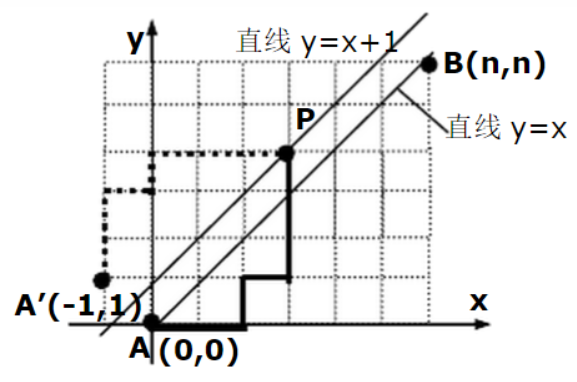## 前置知识

• 排列 (Permutation)
$$P_{n}^m=\frac{n!}{(n-m)!}$$
从 $n$ 个不同的元素里，选出 $m$ 个进行全排列的方案数。

分子 $n!$ 的含义是 $n$ 个元素的全排列，其中有 $n-m$ 个是不需要的，因此他们的顺序没有意义，去掉不同顺序带来的计数，除掉 $n-m$ 的全排列数 $(n-m)!$

• 组合 (Combination)
$$C_n^m=\frac{n!}{m!(n-m)!}$$
从 $n$ 个不同的元素里，选出 $m$ 个组成一个集合的方案数。

相当于排列数中的 $m$ 个元素去掉顺序，即 $P_n^m$ 再除掉 $m$ 的全排列数 $m!$

• 顺序化与去顺序

从排列数和组合数的定义可以看出，排列的顺序带来了更多的方案数；

而组合的选取集合无序性，使得方案数去掉了 $m$ 个元素内部顺序。

我们可以笼统的归纳出：$m$ 个元素有顺序的方案数 $=$ 无顺序的方案数 $\times \ m!$

这样就可以很好地解释一个恒等式：$P_n^m=C_n^m\times m!$

有序与无序的转换可以通过乘或除全排列来实现，这个思想后面我们还会用到。

• 一个组合恒等式
$$C_n^m=C_{n-1}^{m-1}+C_{n-1}^m$$
含义是，从 $n$ 个不同的元素里，选出 $m$ 个组成一个集合的方案，可以由两种情况发展而来：

1. 选上第 $n$ 个元素，前 $n-1$ 个元素中选取 $m-1$ 个。
2. 不选第 $n-1$ 个元素，前 $m-1$ 个元素中选取 $m$ 个。

这种增量的思维，即单独考虑第 $n$ 个元素的选取策略的思考方式很重要，在后面也还会用到。

## 全错位排列

$$D_1 = 0\ ,\ D_2 = 1\ ,\ D_n=(n-1)(D_{n-1}+D_{n-2})\ (n\ge 3)$$

1. 当数字 $k$ 排在第 $n$ 位时，除了 $n$ 和 $k$ 以外还有 $n-2$ 个数，其错排数为 $D_{n-2}$。
2. 当 $k$ 不排在第 $n$ 位时，那么将第 $n$ 位重新考虑成一个新的“第 $k$ 位”，这时的包括 $k$ 在内的剩下 $n-1$ 个数的每一种错排，都等价于只有 $n-1$个数时的错排（只是其中的第 $k$ 位会换成第 $n$ 位），其错排数为 $D_{n-1}$。

## 卡特兰数

\begin{align} H_n=\frac{C_{2n}^{n}}{n+1}=\frac{\prod_{i=n+2}^{2n}i}{n!}\end{align}1. 总共需要走的步数为 $2n$ 步，而向右一共要走 $n$ 步，故总的方案数为 $C_{2n}^{n}$ 。

2. 不合法的方案即为越过 $y=x$ 的路径数，考虑将每一个不合法的方案中从 $(0,0)$ 到第一个越过 $y=x$ 的点这一部分的路径关于 $y=x+1$对称，其余部分不变。我们发现，每一个不合法的路径都一一映射到了一个从 $(-1,1)$ 到 $(n,n)$ 的路径，那么不合法的方案数即为从 $(-1,1)$ 走到 $(n,n)$ 的方案数，因为一共向上要走$n-1$步，向右一共要走$n+1$步，所以不合法的方案数为 $C_{2n}^{n-1}$或 $C_{2n}^{n+1}$。

3. 做一个减法，整理一下就得到了公式：

$$H_n=C_{2n}^n-C_{2n}^{n-1}=C_{2n}^n - C_{2n}^{n}\times \frac{n}{n+1}=\frac{C_{2n}^{n}}{n+1}$$

Catalan 数还有以下几中比较常见的解释：

• $n$ 个 $0$ 与 $n$ 个 $1$ 构成的序列，使得任何一个前缀 $0$ 的个数不少于 $1$ 的方案数。

也就是 16 年高考题的题面啦，把 $0$ 当作向右走一步，把 $1$ 当作向上走一步，就可以解释了。

• 给定 $n$ 对括号，求所有括号正确配对的括号序列数。

把左括号当作向右走一步，把右括号当作向上走一步，就可以解释了。

• 将一个 $n+2$ 条边的凸多边形，通过若干条互不相交的对角线，划分成 $n$ 个三角形的方案数。

这个用组合数学不太好解释，想听的同学找我吧…

## 第二类斯特林数

$$S_n^m=S_{n-1}^{m-1}+m\times S_{n-1}^m$$

• 如果第 $n$ 个元素单独作为一个集合，则方案唯一，剩下的部分组成 $m-1$ 个集合，贡献为 $S_{n−1}^{m−1}$ 。
• 如果第 $n$ 个元素加入之前的集合，那么有 $m$ 个集合可以选择，贡献是 $m\times S_{n-1}^m$ 。

$$S_n^0=0,S_n^1=1,S_n^n=1,S_n^m=0(m>n)$$

n\m 0 1 2 3 4 5
1 0 1 0 0 0 0
2 0 1 1 0 0 0
3 0 1 3 1 0 0
4 0 1 7 6 1 0
5 0 1 15 25 10 1

## 隔板法

1. 每两个相邻的板构成一个盒子。
2. 板是相同的，盒子是不同的。板之间形成的空隙的顺序完成了的对盒子不同的要求，把盒子变成板实质为“去顺序”

## 小球装箱

• 球同，盒子不同，盒子不可以空。

思路是隔板法的第一种，插空法

有 $m$ 个盒子，每两个相邻的板构成一个盒子，所以需要放 $m+1$ 个板。

首先我们在 $n$ 个球的两侧放上两个板，代表左右边缘两个盒子的一侧。

那么剩下就要再插入 $m-1$ 个板，板和板之间不能相邻，也就是每两个球的空隙之间只能放一个板。

有 $n-1$ 个空隙，选出来 $m-1$ 个即可，方案数为 $C_{n-1}^{m-1}$。

• 球同，盒子不同，盒子可以空。

思路是隔板法的第二种，排列法

同样有 $m$ 个盒子，每两个相邻的板构成一个盒子，所以需要放 $m+1$ 个板。

同样首先需要在 $n$ 个球的两侧放上两个板，代表左右边缘两个盒子的一侧。

那么剩下就要再插入 $m-1$ 个板，但这次板和板之间可以相邻，中间不隔着球的两个板代表一个空盒子，每个球球间空隙可以放任意多个板。

这是我们相当于要得到一个包含 $n$ 个球和 $m-1$ 个板的排列，由于球之间相同，板之间相同，所以只需要从 $n+m-1$ 个位置里选出 $n$ 个位置放球即可，方案数为 $C_{n+m-1}^n$，当然也可以从板的角度，写成 $C_{n+m-1}^{m-1}$。

• 球不同，盒子相同，盒子不可以空。

这个问题就是第二类 Stirling 数的定义，将 $n$ 个不同的元素，划分成 $m$ 个不同的非空集合的方案数，所以答案是 $S_n^m$。

• 球不同，盒子相同，盒子可以空。

我们假设有 $i(i\in [1,m])$ 个盒子非空的，由于盒子相同，此时的答案为 $S_n^i$。

由于非空的盒子数不同的情况之间独立，所以答案是 $\sum_{i=1}^mS_n^i$。

• 球不同，盒子不同，盒子不可以空。

此问题相当于是第三个问题的顺序化，也就是原本相同的盒子变成不同的了，需要乘上一个不同顺序带来的情况数，所以按照顺序化的操作得到答案为 $S_n^m\times P_m^m$。

• 球不同，盒子不同，盒子可以空。

球之间的决策是独立的，每个球有 $m$ 个不同的盒子选，不需要考虑是否导致盒子空，方案数为 $m^n$。